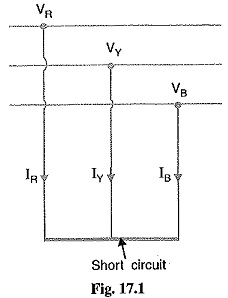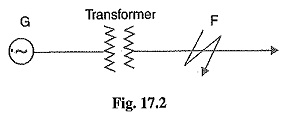## Symmetrical Faults on 3 Phase System:

Most of the Symmetrical Faults on 3 Phase System on the power system lead to a short-circuit condition. When such a condition occurs, a heavy current (called short circuit current) flows through the equipment, causing considerable damage to the equipment and interruption of service to the consumers. There is probably no other subject of greater importance to an electrical engineer than the question of determination of short circuit currents under fault conditions. The choice of apparatus and the design and arrangement of practically every equipment in the power system depends upon short-circuit current considerations.

That fault on the power system which gives rise to symmetrical fault currents (i.e. equal fault cur­rents in the lines with 120° displacement) is called a symmetrical fault.

The symmetrical fault occurs when all the three conductors of a 3-phase line are brought together simultaneously into a short-circuit condition as shown in Fig. 17.1. This type of fault gives rise to symmetrical currents L e. equal fault currents with 120° displacement. Thus referring to Fig. 17.1, fault currents IR, ly and IB will be equal in magnitude with 120° displacement among them. Because of balanced nature of fault, only one phase need be considered in calculations since condition in the other two phases will also be similar.The following points may be particularly noted :

1. The symmetrical fault rarely occurs in practice as ma­jority of the faults are of unsymmetrical nature. How­ever, symmetrical fault calculations are being dis­cussed in this chapter to enable the reader to under­stand the problems that short circuit conditions present to the power system.
2. The symmetrical fault is the most severe and imposes more heavy duty on the circuit breaker.

### Limitation of Fault Current:

When a short circuit occurs at any point in a system, the short-circuit current is limited by the impedance of the system upto the point of fault. Thus referring to Fig. 17.2, if a Symmetrical Faults on 3 Phase System occurs on the feeder at point F, then the short circuit current from the generating station will have a value limited by the impedance of generator and transformer and the impedance of the line between the generator and the point of Symmetrical Faults on 3 Phase System. This shows that the knowledge of the impedance of various equipment and circuits in the line of the system is very important for the determination of short-circuit currents.In many situations, the impedances limiting the Symmetrical Faults on 3 Phase System current are largely reactive, such as trans­formers, reactors and generators. Cables and lines are mostly resistive, but where the total reactance in calculations exceeds 3 times the resistance, the latter is usually neglected. The error introduced by this assumption will not exceed 5%.

Scroll to Top